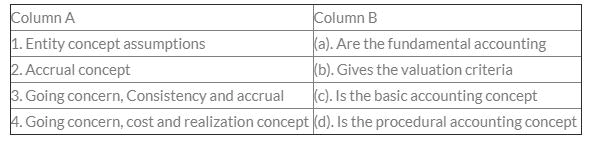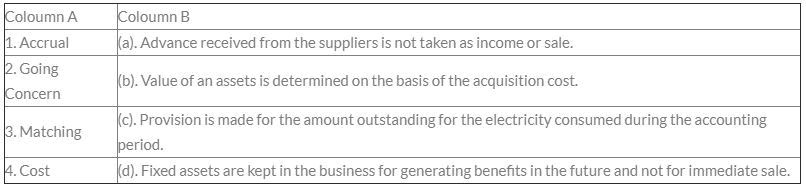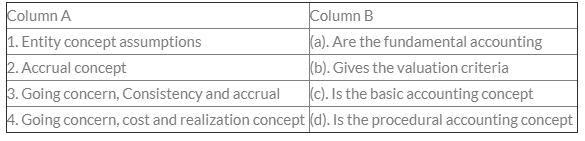Courses

# Test: Accounting Concepts, Principles And Conventions - 4

## 30 Questions MCQ Test Crash Course of Accountancy - Class 11 | Test: Accounting Concepts, Principles And Conventions - 4

Description
This mock test of Test: Accounting Concepts, Principles And Conventions - 4 for CA Foundation helps you for every CA Foundation entrance exam. This contains 30 Multiple Choice Questions for CA Foundation Test: Accounting Concepts, Principles And Conventions - 4 (mcq) to study with solutions a complete question bank. The solved questions answers in this Test: Accounting Concepts, Principles And Conventions - 4 quiz give you a good mix of easy questions and tough questions. CA Foundation students definitely take this Test: Accounting Concepts, Principles And Conventions - 4 exercise for a better result in the exam. You can find other Test: Accounting Concepts, Principles And Conventions - 4 extra questions, long questions & short questions for CA Foundation on EduRev as well by searching above.
QUESTION: 1

Solution:
QUESTION: 2

### A proprietor, Mr. A has reported a profit of Rs. 1,25,000 at the end of the financial year after taking into consideration the following amount: (i). The cost of an assets Rs. 25,000 has been taken as en expense. (ii). Mr. A is anticipating a profit of Rs. 10,000 on the future sale of a car shown as an asset in his books. (iii). Salary of Rs. 7,000 payable in the financial year has not been taken into account. (iv). Mr. A purchased an asset for Rs. 75,000 but its fair value on the date of purchase was Rs. 85,000. Mr. A recorded the value of asset in his books by Rs. 85,000. Q. Which measurement base should be followed in the statement (iv)?

Solution:

The correct option is A

A historical cost is a measure of value used in accounting in which the value of an asset on the balance sheet is recorded at its original cost when acquired by the company. The historical cost method is used for fixed assets under generally accepted accounting principles (GAAP). Since the asset is to be recorded at the previous price ,so historical concept should be followed.

QUESTION: 3

### Match the following items from column A with column BSolution:
QUESTION: 4

The comparison of the financial statement of one accounting period with that of another accounting period is possible only when _______ concept is followed.

Solution:
QUESTION: 5

The proprietor of a business is treated as a creditor for capital introduced by him, according to:-

Solution:
QUESTION: 6

Matching concept means:

Solution:
QUESTION: 7

Which concepts work together for income measurement and recognition of assets and liabilities?

Solution:
QUESTION: 8

X of Delhi purchased a machinery from Y of Chennai for Rs. 10, 00,000. He paid freight of Rs. 2, 00,000 and excise duty of Rs. 1, 00,000. He used the machinery in his business. At the end of the year he charged depreciation Rs. 1, 50,000. The market value of the machine was estimated at Rs. 15, 00,000. At what value the machinery should be shown in the balance sheet at the year end?

Solution:
QUESTION: 9

A proprietor, Mr. A has reported a profit of Rs. 1,25,000 at the end of the financial year after taking into consideration the following amount:

(i). The cost of an assets Rs. 25,000 has been taken as en expense.
(ii). Mr. A is anticipating a profit of Rs. 10,000 on the future sale of a car shown as an asset in his books.
(iii). Salary of Rs. 7,000 payable in the financial year has not been taken into account.
(iv). Mr. A purchased an asset for Rs. 75,000 but its fair value on the date of purchase was Rs. 85,000. Mr. A recorded the value of asset in his books by Rs. 85,000.

Q. Which concept should be followed in the statement (iii)?

Solution:
QUESTION: 10

As per basic accounting Equation Assets = Capital + Liabilities which of the following equation will be true if Ram starts a business with Rs. 5,50,000 & then buy goods worth Rs. 1,50,000 from Madan on credit?

Solution:
QUESTION: 11

According to the prudence concept, closing stock be valued at cost or _____ whichever is less.

Solution:
QUESTION: 12

Money measurement concept

Solution:
QUESTION: 13

If owner’s equity of a business are Rs. 90,000 and profits are of Rs. 60,000 for the year calculate total assets of the business:-

Solution:
QUESTION: 14

Match the following items from column A with column B.Solution:
QUESTION: 15

Business enterprise is a separate identity apart from its owner. Accountant should treat a business as distinct from its owner. This concept is known as:

Solution:
QUESTION: 16

The ‘going concern concept’ is the underlying basis for

Solution:
QUESTION: 17

What is the effect on the Net Assets if cash is received from debtors of Rs. 50,000?

Solution:
QUESTION: 18

Matching concept means:

Solution:
QUESTION: 19

Match the following items from column A with column BSolution:
QUESTION: 20

Human resources can’t be shown in Balance Sheet because of __________ concept.

Solution:
QUESTION: 21

Which is the accounting concept that requires the practice of crediting closing stock in the trading account?

Solution:
QUESTION: 22

Which concept requires that those transactions which can be expressed in terms of money should be recorded in books of account?

Solution:
QUESTION: 23

If owner’s equity of a business are Rs. 90,000 and profits are of Rs. 60,000 for the year calculate total assets of the business:-

Solution:
QUESTION: 24

A proprietor, Mr. A has reported a profit of Rs. 1,25,000 at the end of the financial year after taking into consideration the following amount:

(i). The cost of an assets Rs. 25,000 has been taken as en expense.
(ii). Mr. A is anticipating a profit of Rs. 10,000 on the future sale of a car shown as an asset in his books.
(iii). Salary of Rs. 7,000 payable in the financial year has not been taken into account.
(iv). Mr. A purchased an asset for Rs. 75,000 but its fair value on the date of purchase was Rs. 85,000. Mr. A recorded the value of asset in his books by Rs. 85,000.

Q. What is the correct amount of profit to be reported in the books?

Solution:

The correct option is D.

Profit=125000

Since the asset was taken as expence, we need to add it, and since a future gain is already included it will be subtracted and the salary not taken in account will be subtracted and since the estimated value of assets are the items to be recorded in balance sheet it will not be recorded.

Profit=125000+25000-7000-10000=₹133000

QUESTION: 25

Recording of capital contributed by the owner as liability ensures adherence of principle of

Solution:
QUESTION: 26

What is the objective of conservatism ?

Solution:
QUESTION: 27

A businessman purchased goods for Rs. 25, 00,000 and sold 70% of such goods during the accounting year ended on 31.3.2014. The market value of remaining goods was Rs. 5, 00,000. He valued the closing stock at Rs. 5, 00,000 and not at Rs. 7, 50,000 due to:

Solution:
QUESTION: 28

A proprietor, Mr. A has reported a profit of Rs. 1,25,000 at the end of the financial year after taking into consideration the following amount:

(i). The cost of an assets Rs. 25,000 has been taken as en expense.
(ii). Mr. A is anticipating a profit of Rs. 10,000 on the future sale of a car shown as an asset in his books.
(iii). Salary of Rs. 7,000 payable in the financial year has not been taken into account.
(iv). Mr. A purchased an asset for Rs. 75,000 but its fair value on the date of purchase was Rs. 85,000. Mr. A recorded the value of asset in his books by Rs. 85,000.

Q. Which concept should be followed in the statement (ii)?

Solution:
QUESTION: 29

A trader stated retail business. During the year he sold goods worth Rs. 60,000 and for Rs. 1,20,000 out of which only Rs. 1,00,000 was collected during the year. He had a closing stock of Rs. 10,000. His other business expenses for the period were Rs. 20,000 out of which Rs. 5,000 was outstanding at year end His total profit for the year 2008-09 as per the terms of accrual concept was:

Solution:
QUESTION: 30

Mohan purchased goods for Rs. 15,00,000 and sold 4/5th of the goods amounting Rs. 18,00,000 and met expenses amounting Rs. 2,50,000 during the year, 2011. He counted net profit as Rs. 3,50,000. Which of the accounting concept was followed by him?

Solution: